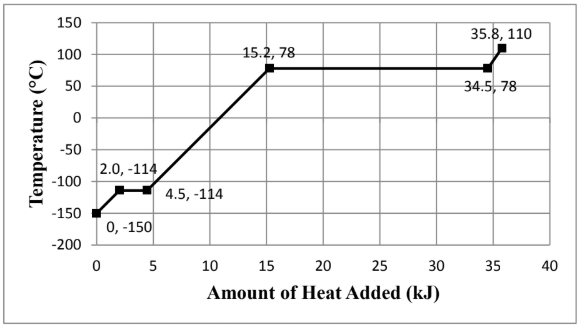# Problem: Consider the heating curve shown below for 22.86 grams of an unknown substance. You may assume constant pressure conditions. Note: the points are labeled as (amount of heat added, temperature). Calculate the heat capacity at constant pressure, C p, (in J/mol∙K) for the gas. Show all work and circle your final answer. The moles were identified to be 0.496 mol.

###### FREE Expert Solution

We are asked to heat capacity at constant pressure, C p, (in J/mol∙K) for the gas

We go through the following steps to solve this problem:

Step 1. Calculate the amount of heat added based on the heating curve

Step 2. Calculate the heat capacity###### Problem Details

Consider the heating curve shown below for 22.86 grams of an unknown substance. You may assume constant pressure conditions. Note: the points are labeled as (amount of heat added, temperature).Calculate the heat capacity at constant pressure, C p, (in J/mol∙K) for the gas. Show all work and circle your final answer. The moles were identified to be 0.496 mol.